# Boundary value problem, numerical methods for partial differential equations

(diff) ← Older revision | Latest revision (diff) | Newer revision → (diff)

Approximate methods of solution which yield the solution to the problem in the form of a numerical table. An exact construction of a solution (in terms of explicit formulas, series, etc.) to a boundary value problem is only rarely possible. The most prevalent of the methods of approximate solution are difference methods (see ); they are applied in the most general problems and are readily computerized. The essential idea of difference methods is to replace the original domain of variation of the independent variables by a discrete set of points, a grid, and to approximate the derivatives figuring in the equation and the boundary conditions by difference quotients at the grid points. As a result of this procedure the original problem is replaced by a system of finitely many algebraic equations (linear or non-linear); this system is known as a difference scheme. The solution of the difference scheme is taken to be an approximate solution of the original problem. The accuracy of the approximation depends on the method of approximation and on the fineness of the grid, i.e. on how densely the grid points fill the original domain. In the rest of this article attention will be restricted to linear boundary value problems for partial differential equations, and it will be assumed that the problem in question is well-posed. In order to justify difference methods one must investigate the well-posedness of the difference problem and its convergence as the grid is refined. A difference problem is said to be well-posed if it has a unique stable solution, whatever the terms on the right-hand sides of the equations. By stability of a difference scheme one means that its solution depends continuously on the right-hand side, uniformly with respect to the grid spacing.

For example, suppose one wishes to solve the Dirichlet problem for the Poisson equation in the square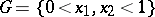with boundary: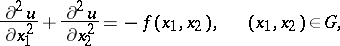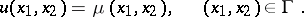The domainis replaced by a square grid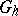with spacing, i.e. by the set of points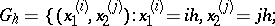with boundaryand the derivatives figuring in the equation by difference quotients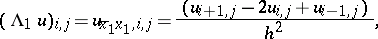where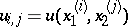. The difference scheme is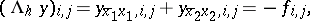(1)whereis the solution.

Problem (1) has a — unique — solution for any inhomogeneous termand any boundary conditions(see ). Moreover, the solution of the difference scheme (1) converges as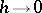to the solution of the original problem in such a way that the scheme is a second-order approximation in the maximum norm, i.e.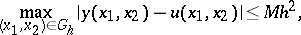whereis a constant independent of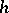.

The difference scheme (1) is a system of linear algebraic equations, characteristically with a large number of equations (to be precise: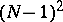equations, with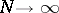as) and a large number of zeros in the matrix of the system; moreover, the system is generally ill-conditioned (the ratio of the minimum eigen value to the maximum one is of the same order of magnitude as,). Such systems of equations, which arise when differential equations are replaced by difference equations, may be solved using various efficient methods, both direct and iterative. Direct methods yield an exact solution of the difference equation after a finite number of arithmetic operations. This category includes various versions of the double-sweep method, including matrix double-sweep, the decomposition method, the fast Fourier transform, and the method of representations by sums (see , , , ). A measure of the efficiency of direct methods is the order of magnitude of the number of operations as. Thus, solving problem (1) by matrix double-sweep requiresoperations, whereas the fast Fourier transform method needs — for the same problem —operations. The most widely used iterative methods for the solution of difference problems are Richardson's method with Chebyshev interpolation points, the alternating-triangular iterative method, and various alternating-direction methods (see ). The efficiency of iterative methods is measured by the order of magnitude of the minimum number of iterations,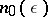, necessary to decrease the error of the initial approximation by a factor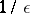. For example, in solving problem (1) by Richardson's method,is of the order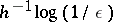, while the alternating-direction method with optimally-selected iteration parameters has. Iterative methods are more universal and simpler to implement than direct methods, and consequently have been widely utilized to solve difference problems.

For example, consider solving the first boundary value problem for the heat equation: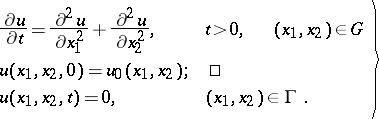(2)

To solve this problem, one prescribes a time grid with spacing: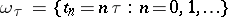and the grid(see above) for the space variables. Let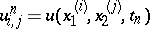. The derivative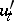is approximated by the quotient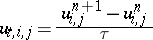and the Laplacianby the difference operator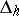. The original equation (2) is replaced by a corresponding difference scheme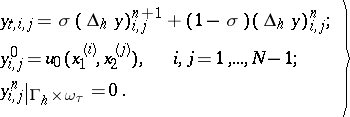(3)

The parameterappearing in these equations determines the stability and accuracy of the scheme. If, the scheme is stable for any grid spacingsand(an absolutely-stable difference scheme). But if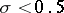, the scheme is stable only if some restriction is imposed on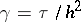(a conditionally-stable difference scheme); for example, the explicit scheme (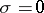) is stable if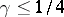. If, the scheme is second-order accurate with respect toand; for other values ofit is first-order accurate inand second-order accurate in. The difference problem (3) is solved "in layers" . The-th layer is the set of all points of the grid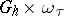for some fixed. The values ofon the zero-th layer (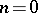) are known from the initial conditions. If the values on the-th layer are known, then the values on the next layer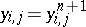are determined from the system of equations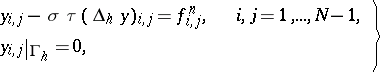(4)

whereThe solution of problem (4) may be found by any of the methods for solving the stationary problem (1). However, there are more economical algorithms for the solution of multi-dimensional non-stationary boundary value problems, namely alternating-direction methods (see ), which enables one to reduce the solution of a multi-dimensional problem to that of a sequence of one-dimensional problems. Thus, the heat equation may be solved using the following alternating-direction scheme: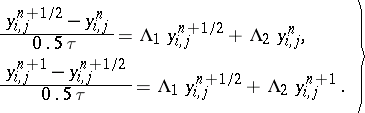This scheme is absolutely stable, second-order accurate and is solved by successive inversion of one-dimensional difference operators.

Even when the difference problem is solved exactly, the solution may well be different — not only quantitatively but also qualitatively — from the solution of the original differential problem. The discrepancy is particularly prominent in dealing with equations the coefficients — or solutions — of which involve singularities (thus, in computing discontinuous solutions of the equations of gas dynamics one usually encounters zones in which the discontinuities are strongly "spread out" ). Thus, direct approximation of a differential problem by a difference problem, when the derivatives are replaced — with a considerable degree of arbitrariness — by difference quotients, does not always yield a good difference scheme. Various principles have been developed for the construction of difference schemes of good quality. Among the successful methods are the balance method and the method of variational-functional approximation (see ). The difference schemes obtained in these methods correctly reflect the integral laws of conservation which are valid for the original equations, and ensure that the relevant difference operators have fixed signs. In the theory of homogeneous difference schemes (see ) one considers questions of constructing difference schemes for equations with variable (including discontinuous) coefficients and investigating their convergence.

How to Cite This Entry:
Boundary value problem, numerical methods for partial differential equations. Encyclopedia of Mathematics. URL: http://encyclopediaofmath.org/index.php?title=Boundary_value_problem,_numerical_methods_for_partial_differential_equations&oldid=14004
This article was adapted from an original article by A.V. Gulin (originator), which appeared in Encyclopedia of Mathematics - ISBN 1402006098. See original article